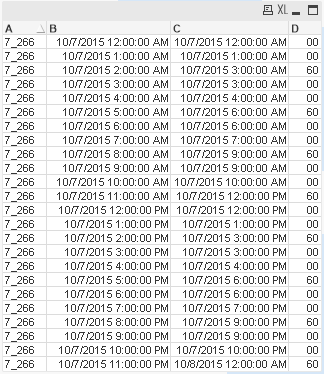QlikView App Development

Discussion Board for collaboration related to QlikView App Development.

Announcements
New Contributor III

Re: How to find the time difference in minutes with respect to different days ?

I meant in this case

Table:

TimeStamp(RangeMax(Temp2, B)) as B,

TimeStamp(RangeMin(Temp, C)) as C,

Interval(RangeMin(Temp, C) - RangeMax(Temp2, B), 'mm') as D;

B,

C,

TimeStamp(Ceil(B + MakeTime(IterNo() - 1), 1/24)) as Temp,

TimeStamp(Floor(B + MakeTime(IterNo() - 1), 1/24)) as Temp2

While Ceil(B + MakeTime(IterNo() - 1), 1/24) <= Ceil(C, 1/24);

LOAD * Inline [

A, B, C

7_266, 10/07/2015 11:30:00 PM, 10/08/2015 7:00:00 AM

];But I guess the issue is that for 10/07 we are getting 30 minutes. But isn't it suppose to be 30 mins from 11:30 to 12:00 is 30 mins or even to 11:59:59 is 30 mins - 1 second. We want to penalize it by the whole minute? I guess I can force it to be 29 mins, but you have to decide if that is correct.

Best,

SunnyMVP

Re: How to find the time difference in minutes with respect to different days ?

It is already showing based on 60 minutes in this case.

New Contributor III

Re: How to find the time difference in minutes with respect to different days ?

But there is a deviation in the result. If i run this specific code. by changing the starting time stamp to  12:00:00 AM the code doesn't provide me with the proper result.

The code used :

Table:

TimeStamp(RangeMax(Temp2, B)) as B,

TimeStamp(RangeMin(Temp, C)) as C,

Interval(RangeMin(Temp, C) - RangeMax(Temp2, B), 'mm') as D;

B,

C,

TimeStamp(ceil(B + MakeTime(IterNo() - 1),1/24)) as Temp,

TimeStamp(floor(B + MakeTime(IterNo() - 1),1/24)) as Temp2,

IterNo() As I

While ceil(B + MakeTime(IterNo() - 1),1/24) <= Ceil(C,1/24);

LOAD * Inline [

A, B, C

7_266, 10/07/2015 12:00:00 AM, 10/08/2015 7:10:00 AM

];

The result for the above code is :The expected result should give me the total minutes within each hour duration.

Suppose 12:00:00 AM - 7:10:00 AM

The result should be

12:00:00 AM-1:00:00 AM - 60 mins

1:00:00 AM-2:00:00 AM - 60 mins

...

...

7:00:00 AM-7:10:00 AM - 10 mins

And then it should stop at 7:10:00 AM. The new rows should not be generated after 07:10:00 AM.

New Contributor III

Re: How to find the time difference in minutes with respect to different days ?

Sorry the time stamps are 10/07/2015 12:00:00 AM, 10/07/2015 7:10:00 AM.

Attaching the file.MVP

Re: How to find the time difference in minutes with respect to different days ?

Try this:

Table:

TimeStamp(RangeMax(Temp2, B)) as B,

TimeStamp(RangeMin(Temp, C)) as C,

Interval(RangeMin(Temp, C) - RangeMax(Temp2, B), 'mm') as D;

B,

C,

TimeStamp(Ceil(If(B = Round(B, 1/24), B + 1/24, B) + MakeTime(IterNo() - 1), 1/24)) as Temp,

TimeStamp(Floor(B + MakeTime(IterNo() - 1), 1/24)) as Temp2

While Ceil(B + MakeTime(IterNo() - 1), 1/24) <= Ceil(C, 1/24);

LOAD * Inline [

A, B, C

7_266, 10/07/2015 12:00:00 AM, 10/07/2015 7:10:00 AM

];

Highlighted
New Contributor III

Re: How to find the time difference in minutes with respect to different days ?

Sorry for the delay in replying back.

Sunny, i think you should look at the entire data which i'm using for which i'm applying the code.There are some deviations.

There are minutes in negative.

In one particular case %key-296. There is a mismatch in From & To date time calculation and also the minutes calculation.MVP

Re: How to find the time difference in minutes with respect to different days ?

So basically you want to get rid of any negative times, right? Try this:

Table:

TimeStamp(RangeMax(Temp2, B)) as B,

TimeStamp(RangeMin(Temp, C)) as C,

Interval(RangeMin(Temp, C) - RangeMax(Temp2, B), 'mm') as D

Where RangeMin(Temp, C) - RangeMax(Temp2, B) > 0;

B,

C,

TimeStamp(Ceil(If(B = Round(B, 1/24), B + 1/24, B) + MakeTime(IterNo() - 1), 1/24)) as Temp,

TimeStamp(Floor(B + MakeTime(IterNo() - 1), 1/24)) as Temp2

While Ceil(B + MakeTime(IterNo() - 1), 1/24) <= Ceil(C, 1/24);